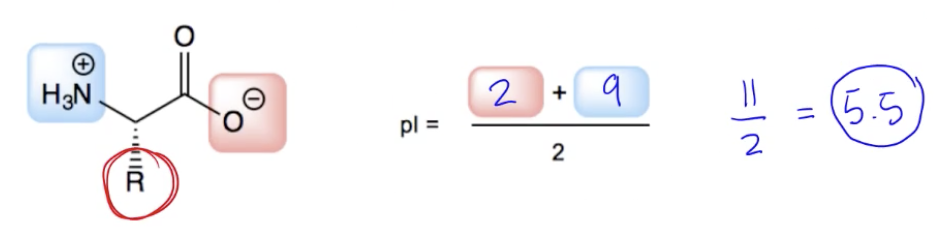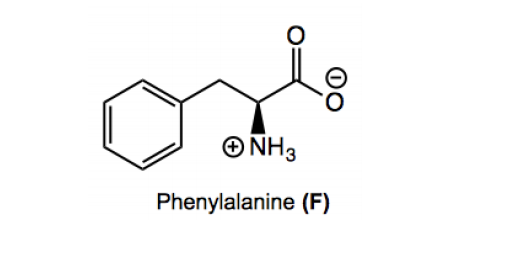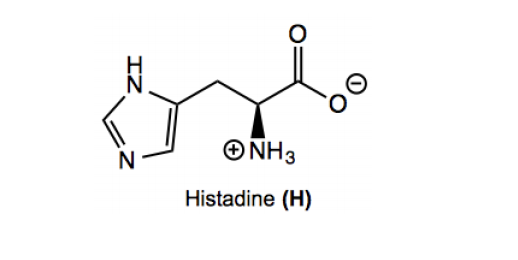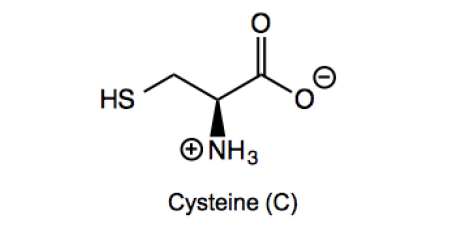Start typing, then use the up and down arrows to select an option from the list.## Organic Chemistry

Learn the toughest concepts covered in Organic Chemistry with step-by-step video tutorials and practice problems by world-class tutors.

26. Amino Acids, Peptides, and Proteins

In this section we will be doing small calculations in order to determine the isolectric point (pI) of various amino acids.

1
concept

## Definition of Isoelectric Point3m
Play a video:2
concept

## Isoelectric Point of Non-Charged Amino Acids3m
Play a video:
so all 20 amino acids fall into three different buckets of ways to calculate the ice ice Electric point. I just wanna look at what those categories are. First, the first category is non acidic or basic amino acids. That's group one. Group two is acidic and basic amino acids, and Group three is just Sistine. Sistine is its own exception, so it's gonna be in its own bucket. So what that means is that if we were to work backwards from this, the ice electric point of Sistine Sistine is in its own group, the ones that are in the acidic and basic amino acids group. What are they? Well, we should have that memorized. We should know that the acidic ones R E d. And we should know that the basic ones are K h R. Right. So that means that if my amino acid is in that bucket, I use this technique. If it's in this bucket, I used this technique. So that means that what types of amino acids are in the first bucket, almost all of them. So I'm just gonna put here everything or I'm just gonna put here all minus e the okay age are and see. Okay, so all of them, all 14 of them, besides those will be in this first bucket. So let's talk about that first category. So if you have one of the immuno acids that's not in these special categories, you could just calculate it as a generic amino acid. Which is the best part. All you do is you just look up the PK a values and average that means 14 of are really straightforward. So federal Allan E. Is that one in this first group. Yes, because it's not acidic. It's not basic. It's not assisting. So what I do is I have to look up the exact values for federal Valentine. Let's go ahead and do that. Now. What I see is that my values are, um, 1.83 and 9.13 So let's go ahead and write those down. So it was 1.93 and nope, 8.1 point 83 and nine 0.13 I almost got that wrong. Okay, Dyslexia. You did not win. So, um so basically, those are my two values. So here we're just gonna have to use a calculator in average them. What that means is that I'm going to add 1.83 plus 9.1 3/2. And whatever that's equal to will be the answer. So I'm just gonna calculate it right now on my phone. So 1.83 plus 9. equals 10.96 divided by two equals that the Ice Electric a point of federal Allen is Cool, awesome. So let's go ahead and do the next one.3
concept

## Isoelectric Point of Charged Amino Acids2m
Play a video:
in this next category, we have acidic and basic side chains, and these are gonna have to use a different rule. And the reason is because you can't just average them. There are now three ionized able groups, and when you have three, you don't just average three of them. You still are only supposed to use to. So how do you pick the two that you're supposed to use? Well, what you do is you pick the two similar groups. You look for the two most similar groups and then those of the PKK's that you use. Now, I already drew out the three p. K. S for you that I got from the sheet. But just be aware that we're only gonna use two of these. One of these is gonna get scratched out. It's not gonna get used for ice Electric point. So the place that you always start is with the side chain because the side chain is always gonna be one of your two. So let's circle aside, chain. Highlight it for sure. I'm gonna use this peak A. That PK is six. So for sure, that's gonna be one of them. But now I have to decide. Is the nitrogen group more similar? Or is the oxygen group more similar to the history and side chain? And the answer is the nitrogen. Okay, Why guys just has to do with Adams. It's that easy. If you have nitrogen in your side chain, you pick the ammonium to be the most similar. If you have oxygen's in your side chain, you pick the oxygen to be most similar. So if this had been glue tannic acid that has carb oxalic acids on both sides, you pick the O's. But if this was Argentine, which has nitrogen on one side, then you pick the nitrogen and the nitrogen is being similar. Okay, In this case, history in has what? Nitrogen? So I would pick the nitrogen group. Okay. What that means is that I'm going to use this as my second group, so I'm gonna use this. PK is my second p a. And I'm scratching out PK one because I'm not gonna use it. That's not the most similar. And now we average these out. So this part's easy 6.0 plus 9.17 divided by two. So we're just gonna use your calculator again. Six plus 9.17 equals 15. 17. Divided by two equals at the is electric point of histamine is 7.59 I'm rounding up. Okay, Awesome. So we're done with this one. Let's move on to the next category.4
concept

## Isoelectric Point of Cysteine1m
Play a video:
so technically Sistine doesn't need to have its own category. But I put it here because I would. I realized that could be the most confusing one. And the reason is because Sistine is the on Li Ion Izabal side chain that has neither an oxygen or nitrogen, right? It's the only one that has a hetero atom that's different. So you could be really confused trying to pick which one similar. It's the same rule, but you might just have a hard time applying it, you might say. Well, is the sulfur more like the nitrogen or is it more like the oxygen? I just wanted to make it clear to you guys that for the purpose of this exercise, it is more similar to the oxygen. And it makes sense because, remember, the oxygen is either gonna have ah, hydrogen or a negative charge, right? Same thing with the sulfur. It's either gonna have a hydrogen or a negative charge is never gonna have to H is in a positive. Okay, so this is just basically an extra little point to say that you average the sulfur and the oxygen as the ones being similar. It's basically the same thing as the acid in based rule is just a different application of it. Okay, So that means that the PKK's that I'm using here. Well, I'm gonna use these two groups, which means the PKs. I'm using our PK one and PK three. And I'm not using p k two. Okay, so now we're just gonna add them together. So it's gonna be 196 plus 818 over to. So I'm gonna do that in my calculator. So I'm getting 10. 14. Divided by two equals a isil electric point of 5.0 seven. Cool. Awesome guys. So we're done with this page. Let's move on to the next.5
Problem

Calculate the isoelectric point of tyrosine (Y)